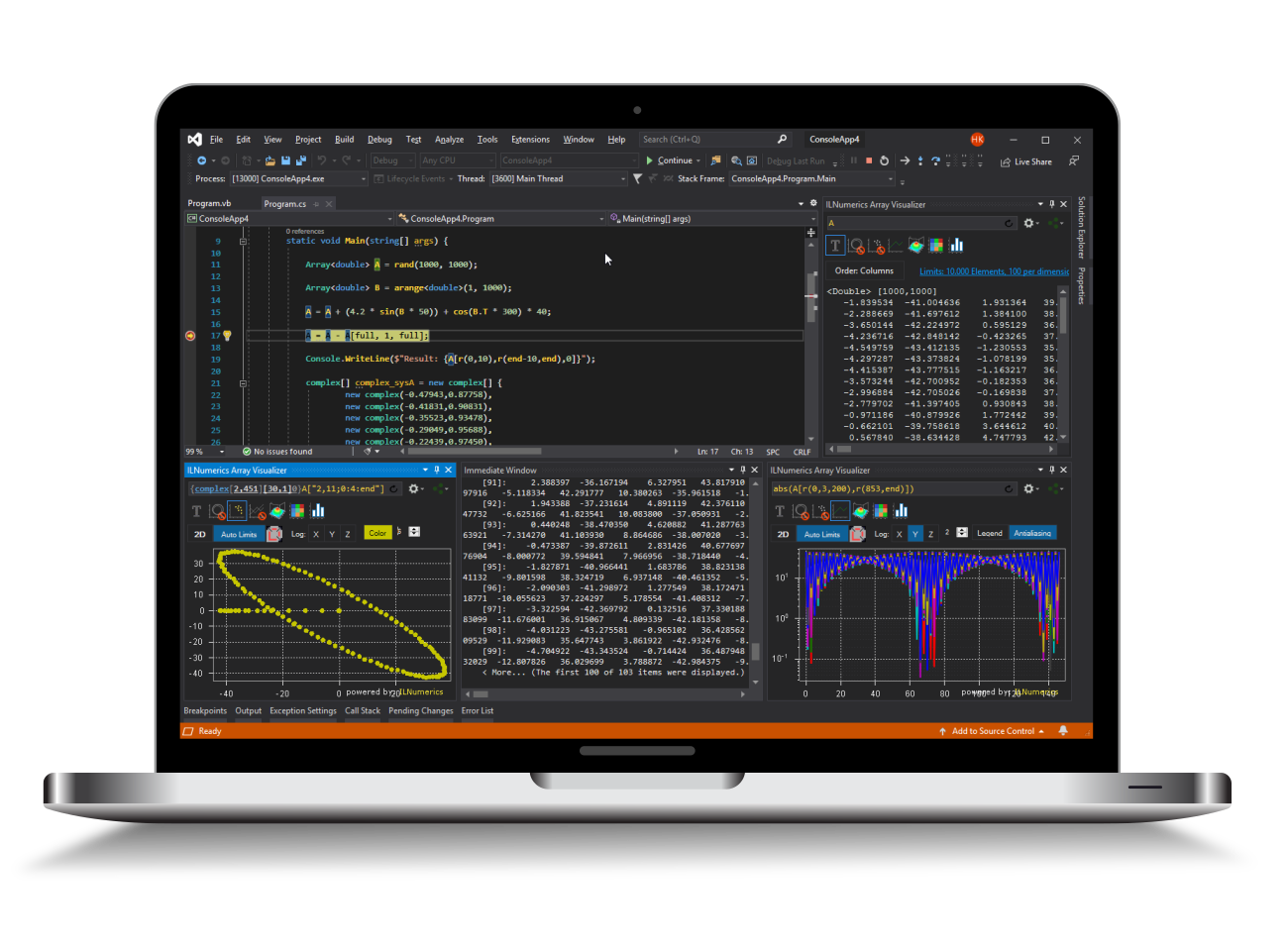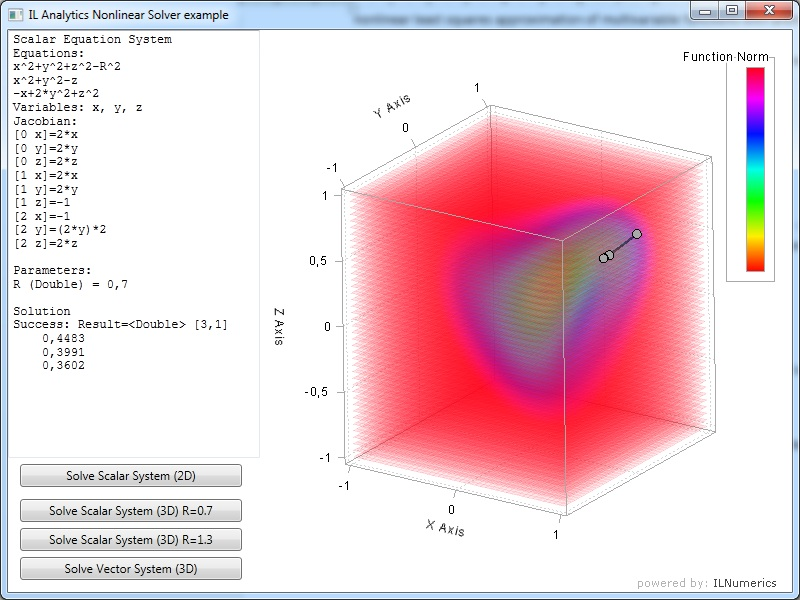Industrial Data Science
in C# and .NET:
Simple. Fast. Reliable.ILNumerics - Technical Computing

Modern High Performance Tools for Technical

Computing and Visualization in Industry and Science

tgt

# ILAnalytics - Symbolic Math for ILNumerics (open source)

ILAnalytics is a free open source plugin for ILNumerics computational library by Sergey L. Gladkiy. The main objective of the ILAnalytics project is to combine the powerful capabilities of numerical methods implemented in the ILNumerics library with the convenience of analytical expression manipulation provided by the ANALYTICS library. ILAnalytics core assembly realizes the integration between the analytical evaluation engine and ILNumerics array data. The project includes four modules: Evaluation, Solution, Optimization and Approximation. The Evaluation library implements most of the mathematical operations and functions for double and complex arrays defined in ILNumerics. The Solution and Optimization assemblies contain special classes for solving nonlinear equation systems and optimization problems defined in analytical form. The Approximation library implements high level classes for linear and nonlinear approximations with arbitrary basis functions set up as analytical expressions.

The advantages of using analytical capabilities are:

• Writing code without special classes for calculation delegates
• Easy storage and transmission of data as strings
• Convenient data presentation for developer and user
• Automatically calculated derivatives for numerical solvers
• Parameterized problem solution

## ILAnalytics Evaluation

The Evaluation assembly contains the realization of a special Translator class – ILTranslator. This class registers analytical operations with ILNumerics double and complex arrays.  Registered operations include:

• Standard algebraic operators (+, -, *, /, ^)
• Special vector and matrix operators (dot product, transposition)
• Transcendental functions (sin, cos, exp, log and so on)
• Basic special functions (abs, sign, ceil and so on)
• Special functions (Bessel, gamma, error and so on)
• Linear algebra functions (LU decomposition, eigenvalues calculation and so on)
• FFT operations

## ILAnalytics Solution

ILAnalytics provides analytical calculated Jacobians for nonlinear solvers that can improve the convergence process due to more precise data calculation.  Also, the equation system is parametric – it contains ‘R’ parameters that can be changed between solutions (without recreation of the system data). So, the system can be solved sequentially for different parameter values.

Advantages of using analytical equation system solvers:

• No need to write special classes for calculation delegates
• Easy storage of data as strings (no need to make storage system for delegates)
• More convenient data presentation for developer and user
• Automatically calculated derivatives for numerical solvers
• Parametrized system solutions## ILAnalytics Optimization

The Optimization assembly provides possibilities of solving nonlinear optimization problems, set up in analytical form, with numerical solvers provided by ILNumerics.## ILAnalytics Approximation

The Approximation extension allows using ILNumerics approximation algorithms with arbitrary basis set ups in the form of analytical expressions. The main functionality is:

• Linear least squares approximation
• Nonlinear least squares approximation
• Constructed linear and nonlinear multivariable bases of any order
• Predefined linear and nonlinear 1D and 2D bases (exponential, polynomial, Fourier)